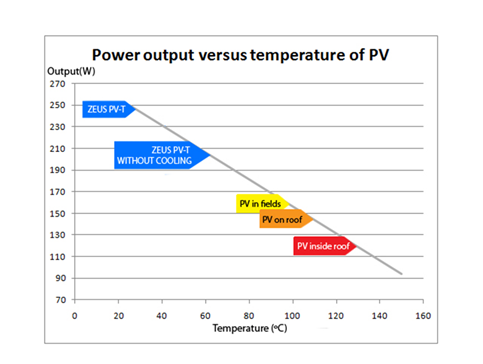﻿ PV loss calculator.xls
 PV vs. TEMPERATURE LOSS CALCULATOR PV Specs: Watts peak: WTemperature Coefficient %/K: % Number of panels: Total kW/h: kW/h Operation Data: Operation Temperature(°C): Operation Hours/Day: hours Financial Data: Cost/kWatt: kW/h performance: kW/h Lost: kW/h \$ Income/Hour: \$ Loss/Hour: \$ Income/Day: \$ Loss/Day: \$ Income/Week: \$ Loss/Week: \$ Income/Month: \$ Loss/Month: \$ Income/Year: \$ Loss/Year: